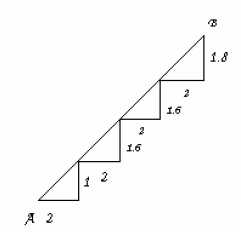## Find the straight distance between a and b, Mathematics

Assignment Help:

There is a staircase as shown in figure connecting points A and B. Measurements of steps are marked in the figure. Find the straight distance between A and B. (Ans:10)Ans:  Apply Pythagoras theorem for each right triangle add to get length of AB.

#### Find the coordinates of the other two vertices, The two opposite vertices o...

The two opposite vertices of a square are (-1, 2) and (3, 2). Find the coordinates of the other two vertices.

what is 5+10

#### Project, elliptical path of celestial bodies

elliptical path of celestial bodies

#### A fire in a building b is reported on telephone, A fire in a building B is ...

A fire in a building B is reported on telephone to two fire stations P and Q, 10km apart from each other on a straight road.  P observes that the fire is at an angle of 60 o to th

#### The ratio of boys to girls at the dance was 3:4, The ratio of boys to girls...

The ratio of boys to girls at the dance was 3:4. There were 60 girls at the dance. How many boys were at the dance? Use a proportion comparing boys to girls at the dance. Boys/

#### Example of a function - inflection point, 1. (a) Give an example of a funct...

1. (a) Give an example of a function, f(x), that has an inflection point at (1, 4). (b) Give an example of a function, g(x), that has a local maximum at ( -3, 3) and a local min

#### Randomly chosen boy can run this race in 302 sec, School run known to posse...

School run known to possess normal distribution with mean 440 sec & SD 60 sec. What is probability that randomly chosen boy can run this race in 302 sec.

#### Geometria, un prisma retto ha per base un rombo avente una diagonale lunga ...

un prisma retto ha per base un rombo avente una diagonale lunga 24cm. sapendo che la superficie laterale e quella totale misurano rispettivamente 2800cm e3568cm ,calcola la misura

#### Sketch a graph of the microphone signal, Figure shows noise results for a p...

Figure shows noise results for a prototype van measured on a rolling road. The vehicle had a four-cylinder-in-line engine. The engine speed was varied in 3rd gear from just above

#### Undamped - forced vibrations, We will firstly notice the undamped case. The...

We will firstly notice the undamped case. The differential equation under this case is, mu'' + ku  = F(t) It is just a non-homogeneous differential equation and we identify h